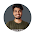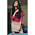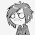# Class IX, Solutions of Ncert Cbse Mathematics - Cbse Notes and Answers of Math Sample Questions

## CBSE Board - Class 9, NCERT Mathematics

### NCERT Solutions and CBSE Guide

#### Tips for Easy Math Solutions

 NCERT Math Chapter & Exercises Class IX Ncert Cbse  Math Solutions NUMBER SYSTEM Exercise 1.1 (Page 5) Exercise 1.2 (Page 8) Exercise 1.3 (Page 14) Exercise 1.4 (Page 18) Exercise 1.5 (Page 24) Exercise 1.6 (Page 26) POLYNOMIALS Exercise 2.1 (Page 32) Exercise 2.2 (Page 34, 35) Exercise 2.3 (Page 40) Exercise 2.4 (Page 43, 44) Exercise 2.5 (Page 48, 49, 50) COORDINATE GEOMETRY Exercise 3.1 (Page 53) Exercise 3.2 (Page 60, 61) Exercise 3.3 (Page 65) LINEAR EQUATIONS IN TWO VARIABLES Exercise 4.1 (Page 68) Exercise 4.2 (Page 70) Exercise 4.3 (Page 74, 75) Exercise 4.4 (Page 77) INTRODUCTION TO EUCLID’S GEOMETRY Exercise 5.1 (Page 85, 86) Exercise 5.2 (Page 88)
The Table contines after the Ad -

 LINES AND ANGLES Exercise 6.1 (Page 96, 97) Exercise 6.2 (Page 103, 104) Exercise 6.3 (Page 107, 108) TRIANGLES Exercise 7.1 (Page 118, 119, 120) Exercise 7.2 (Page 123, 124) Exercise 7.3 (Page 128) Exercise 7.4 (Page 132) QUADRILATERALS Exercise 8.1 (Page 146, 147) Exercise 8.2 (Page 150, 151) AREAS OF PARALLELOGRAMS AND TRIANGLES Exercise 9.1 (Page 155) Exercise 9.2 (Page 159, 160) Exercise 9.3 (Page 162, 163, 164) Exercise 9.4 (Page 164, 165, 166) CIRCLES Exercise 10.1 (Page 171) Exercise 10.2 (Page 173) Exercise 10.3 (Page 176) Exercise 10.4 (Page 179) Exercise 10.5 (Page 184, 185, 186) Exercise 10.6 (Page 186, 187) CONSTRUCTIONS Exercise 11.1 (Page 191) Exercise 11.2 (Page 195) HERON’S FORMULA Exercise 12.1 (Page 202, 203) Exercise 12.2 (Page 206, 207) SURFACE AREAS AND VOLUMES Exercise 13.1 (Page 213) Exercise 13.2 (Page 216, 217) Exercise 13.3 (Page 221) Exercise 13.4 (Page 225) Exercise 13.5 (Page 228) Exercise 13.6 (Page 230, 231) Exercise 13.7 (Page 233) Exercise 13.8 (Page 236) Exercise 13.9 (Page 236, 237) STATISTICS Exercise 14.1 (Page 239) Exercise 14.2 (Page 245, 246) Exercise 14.3 (Page 258, 259, 260, 261) Exercise 14.4 (Page 269) PROBABILITY Exercise 15.1 (Page 283, 284, 285)

##### 16 comments:
Write comments
1.this is not opening i cannot find my maths solutions

2.wht am i not getting the solutions only few of them are there........

3.some of them are not available
please make it available

4.this is not open plz available it

5.plz make them available

6.plz plz plz make them avalible its urgent..!! i wann all the ans plzzzzzzzz.....

7.why all exercises are not available?

8.I could't find my maths solutiom.Plzzzz..... Provide solution of all exercise.
Md.kaish ansari
Class-9
School-G. M. ACADEMY, SALEMPUR,DEORIA,U.P.
Affiliated to CBSE,NEW DELHI
Affiliation No.-2131187

9.what is this yaa...... some lessons are not at all opening... then... how i can READ.... ???

10.what is this yaa... i cannot open some chapters also... what to do..???

11.waste of time!!!!!!!!!11

12.If you please add the solutions of chapter 9,exercises pleeeeeeese !

13.what is this? add the solution

14.this is just rubbish... u plz post the solutions of circles ex10.6 and vol&surface areas

15.what the hhhhhheeeeelllllllll!!!!!!!!!!!!!!!!!!!!!!....................................i can't find ex-11.2 solutions!!

16.What the hell is that new interface, I don't like it. It is fully stinky interface , the previous inerface is much attractive than this one